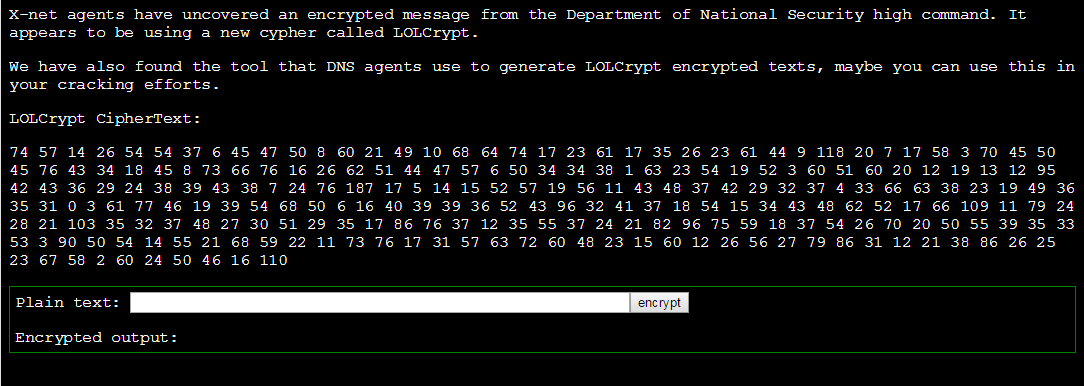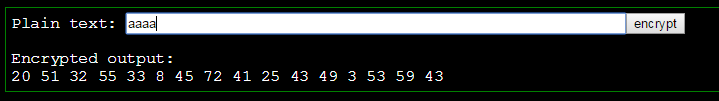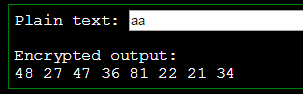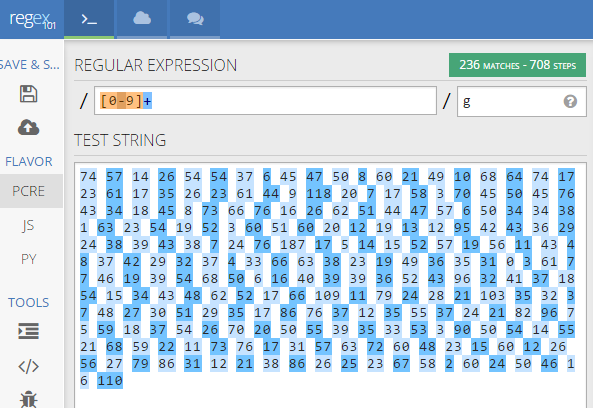# EFF-CTF 2016 - LOLCrypto - Crypto Challenge

## January 27, 2016

Reading time ~1 minute

I have a guest writeup this week for the EFF-CTF we did. Welcome Steven who I worked with to solve the EFF-CTF this week which was part of Enigma 2016 security conference.  Take it away Steven:

Level0x3 for the EFF-CTF required cracking homebrew crypto. The level was as follows:At first we tried “aaaa” as our input as a test.Interesting, lets make sure it’s deterministic by checking the same input again.hmm, everything changed.

We then tested a single character to see what the length of the output would be.It’s looking as if one character becomes 4 numbers when encrypted. Lets test that theory:“aa” becomes 8 numbers, so we are on the right path.

I had the idea to add the numbers up and see if them came to the same summation. Which they did! If that failed, I was going to check if some mathematical operation of the 4 numbers (or part of) would be the same. Failing that, perhaps one or more of the numbers were decoys and discarded when decrypted.

• 67+12+2+77 = 158
• 30+56+48+24 = 158
• 65+7+56+30 = 158
• 52+20+54+32 = 158

Yep! So, our theory is groups of 4 numbers add to the same sum. Lets make sure our ciphertext that we need to crack has groups of 4.

I used https://regex101.com with /[0-9]+/gPerfect.

We can now use a chosen plain text attack, so we encrypt “abcdefghijklmnopqrstuvwxyzABCDEFGHIJKLMNOPQRSTUVWXYZ._ :1234567890′!”

We can then can map the output to each character in that list.

Kris created a script in Python that could do that for us:

``````

#!/usr/bin/python

c = map(int, open('ciphertext.txt', 'r').read().split())
alphacipher = map(int, open('alphabetciphered.txt', 'r').read().split())

alphabet = list("abcdefghijklmnopqrstuvwxyzABCDEFGHIJKLMNOPQRSTUVWXYZ._ :1234567890'!")

# Generate a translation table
translation = []
for i in range(,len(alphacipher),4):
j = alphacipher[i] + alphacipher[i+1] + alphacipher[i+2] + alphacipher[i+3]
translation.append(j)

# decipher ciphertext
plaintext = []
for i in range(,len(c),4):
j = c[i] + c[i+1] + c[i+2] + c[i+3]
plaintext.append(alphabet[translation.index(j)])

print "[+] Plaintext: " + "".join(plaintext)

``````

Which when we run, gives us the flag:

``````root@kalimate:~/eff# python dec2.py
[+] Plaintext: This week's decryption passphrase is: Don't BULLRUN me bro!

``````

### niteCTF - CBC-Jail

A unique combination of Python jailbreak and crypto flaw that had me learning a lot about AES-CBC mode. Super fun for me to get this solu...… Continue reading

#### niteCTF - Rabin to the Rescue

Published on December 12, 2021

#### HTB CyberSanta 2021 - Crypto Writeups

Published on December 04, 2021